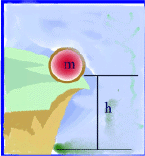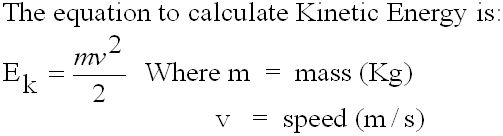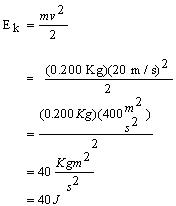#### Potential & Kinetic Energy

Energy is defined as the ability to do work.

Energy can take many forms.

In mechanics the two most common forms of energy are Kinetic Energy  and Potential Energy.

Potential Energy is the energy stored in an object when it has been lifted off the ground.

For example, in the illustration below a red ball is about to fall off a cliff.  Before it falls off the top of the cliff, it has stored potential energy.An object with mass m (in kilograms), lifted up at a height of h meters above ground, against gravity, will have stored gravitational potential energy.

The symbol for Potential Energy is P.E. or Ep

The equation to calculate Poential Energy is:

P.E. = m x g x h

The units of Potential Energy, are the same as the units of work.
Work and energy are both calculated in JOULES.

Example 1:
In the picture above, the ball on the cliff has a mass of 300 g and the cliff is 20 meters above the ground.  What is the potential energy of the ball?

Solution:
Given: m = 300 g = 0.300 Kg       Find: P.E.       P.E. = m x g x h
h = 20 m                                                            = (0.300 Kg)((9.8 N/Kg)(20 m)
g = 9.8 K/Kg                                                     = 58.8 Nm = 58.8 J

Kinetic Energy is the energy of a moving object.

As the red ball in the above example falls off the topof the cliff, it will release its potentialn energy and acquire the energy of an object in moion, called kinetic energy.

The symbol for Kinetic Energy is K.E. or Ek

The kinetic energy of an object depends on the mass (m) of the object and on the speed (v) of the object.Example 2:

A 200 g soccer ball is kicked and moves with a speed of 20.0 m/s.

Calculate its kinetic energy.

Solution:

Find K.E. (or Ek)
Given: m = 200 g = 0.200 Kg
v = 20 m/sThe soccer ball has a kinetic energy of 40 J